# DAYS360 Function

Calculate the number of days between two dates based on a 360-day year

## What is the DAYS360 Function?

The DAYS360 Function in Excel is categorized under Excel Date/Time functions. This function helps to calculate the number of days between two dates, based on a 360-day year.

As a financial analyst, the DAYS360 Function in Excel becomes useful in preparing reports such as an Aging schedule for debtors or computing a payments schedule for accounting systems that are based on twelve 30-day months.

### Formula

=DAYS360(start_date,end_date,[method])

The DAYS360 function includes the following arguments:

1. Start_date – This is a required argument. It is the start of the period.
2. End_date – This is a required argument. It is the end of the period.
3. Method – This is an optional argument. It is a Boolean value. Here we specify the method to be used in the calculation. The method can either be:
1. False or omitted – US (NASD) method used. Under this method:
• When the starting date is the last day of a month, it is set as the 30th day of the same month.
• Whereas, when the ending date is the last day of a month then:
1. If the start date is the last day of the month, the end date is set to the 1st of the following month.
2. Otherwise, the end date is set to the 30th of that month.
2. True – European method used. Here, the starting dates and ending dates that occur on the 31st day of a month become equal to the 30th day of the same month.

The DAYS360 function will return a numerical value.

### How to use the DAYS360 Function in Excel?

To understand the uses of the DAYS360 function and how it can be used, let’s consider a few examples:

#### Example 1

Suppose we are given the following dates in text format. We will use the function to see what results are produced: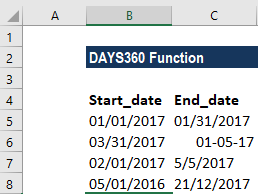Using the DAYS360 function, we get the following results under different scenarios: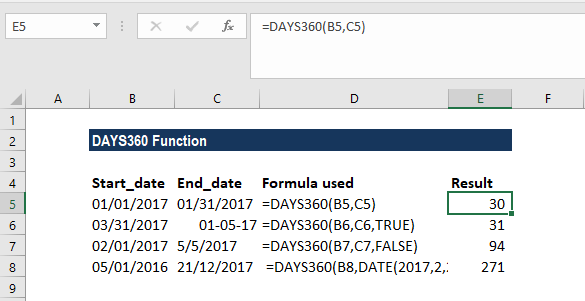The formula used in D2 and D4 omitted the method argument so this function used the US (NASD) method.

Whereas, in D3, we set the method argument as TRUE, so this function uses the European method. If we take the same dates as shown below, we will understand that DAYS360(A2,B2) and DAYS360(A2,B2,TRUE) will give different results, as shown below: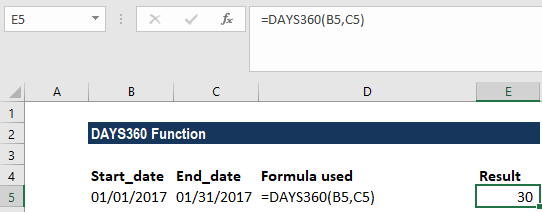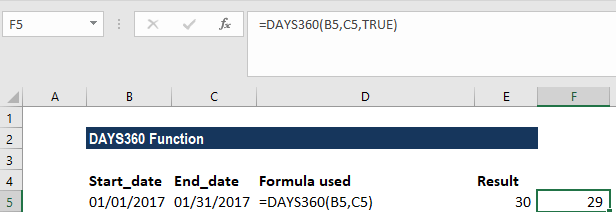Remember that the start_date and end_date arguments that were supplied should either be cell references or values returned from functions (in cell D4, the DATE function is used to supply the end_date argument).

The DAYS360 function calculates the difference between two dates and tells us the number of days. It assumes that there are 30 days in each month. The 30-day assumption is important in calculating the year difference between two dates.

#### Example 2

Suppose we are given the dates as:

• Start date: January 1, 2016
• End date: June 2, 2017

Let’s assume we wish to find out the year difference between two given dates using this function. The formula to be used would be =DAYS360(DATE(2016,1,1),DATE(2017,6,2))/360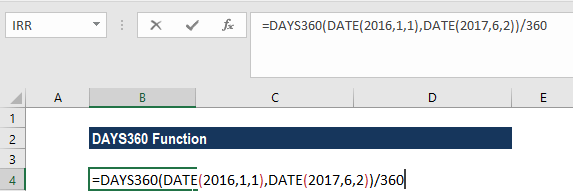We get the following result: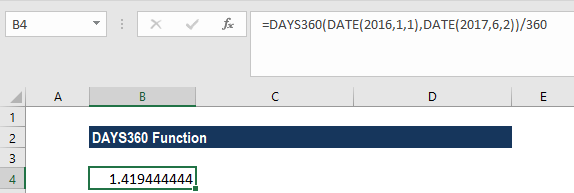The result is the year difference between the two dates. On a similar basis, we can find out the difference in months.

### A few notes about the DAYS360 Function

1. #NUM! error – This occurs when the start_date and the end_date arguments are numerical values but are not recognized as valid dates.
2. #VALUE! error – This occurs when the:
• One or both of the given arguments – start_date or end_date – are text values that cannot be interpreted as dates.
• The arguments given are non-numeric.

Thanks for reading CFI’s guide to important Excel functions! By taking the time to learn and master these functions, you’ll significantly speed up your financial analysis. To learn more, check out these additional CFI resources:

• Excel Functions for Finance